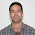## Sunday, February 05, 2017

### The Hardness of Reals Hierarchy

In my last post (here) I defined the following hierarchy (which I am sure is not original- if someone has a source please leave a comment on it)

Z_d[x] is the set of polys of degree d over Z (the integers)

ALG_d is the set of roots of these polys.

ALG_1 = Q (The rationals)

Given a real alpha I think of its complexity as being the least d such that alpha is in ALG_d. This is perhaps a hierarchy of hardness of reals (though there are an uncountable number of reals that are NOT in any ALG_d.)

I then said something like

Clearly

ALG_1 PROPER SUBSET ALG_2 PROPER SUBSET ALG_3 etc.

But is that obvious?

Clearly''  2^{1/d} is not in ALG_{d-1}. And this is not a trick- YES 2^{1/d} is NOT in ALG_{d-1} But how would you prove that. My first thought was `I'll just use Galois theory' And I am sure I could dust off my Galois theory notes (I had the course in 1978 so the notes are VERY dusty) and prove it. But is there an elementary proof. A proof a High School Student could understand.

How to find out? Ask a bright high school student to prove it! Actually I asked a Freshman Math Major who is very good, Erik Metz. I thought he would find a proof, I would post about it asking if it was known, and I would get comments telling me that OF COURSE its known but not give me a reference (have I become cynical from  years of blogging. Yes.)

But that's not what happened. Erik had a book Problems from the Book by Dospinescu and Andreescu that has in it a lovely elementary proof (it uses Linear Algebra) that 2^{1/d} is NOT in ALG_{d-1}.

Hence the hardness of reals hierarchy is proper. For a write up of just the proof that
7^{1/3} is not in ALG_2 (which has most of the ideas) see this writeup

1.Comment on your write-up: Why is your equation $a_2 7^{7/3} + a_1 7^{1/3} + a_0$? Shouldn't it be $a_2 7^{2/3} + a_1 7^{1/3} + a_0$ instead?

1.Typo/fixed/thanks

2.How can 2^(1/d) not be in ALG_d, it is a root of X^d-2...

1.Typo/fixed/thanks

3.Nice, I haven't seen this method! Here's another (similar) elementary approach, for students familiar with polynomial division but not linear algebra.

Step 1: the least-degree integer polynomial P satisfied by 2^(1/d) would have to divide A = x^d-2, where the quotient Q has rational coefficients (proof: dividing A into P leaves a remainder with degree smaller than A).

Step 2: In fact, we can arrange for P and Q to _both_ have integer coefficients (proof: Gauss's lemma, really just a divisibility computation with polynomial coefficients).

Step 3: But x^d-2 is irreducible over the integers (proof: Eisenstein's criterion, a divisibility argument very similar to Step 2).

The statement of Eisenstein's criterion usually encompasses both Steps 2 and 3 (and then cites Gauss's lemma in the proof), but it's nice to separate these out more explicitly as above, since one provides a nice warmup for the other. Full details are in their respective Wikipedia articles:

https://en.wikipedia.org/wiki/Eisenstein's_criterion
https://en.wikipedia.org/wiki/Gauss%27s_lemma_(polynomial)

1.Yeah indeed it can be proved in almost the same way that the reals that belong to ALG_d and not to ALG_{d-1} are exactly those that are roots of an irreducible polynomial of degree d :) Nice result to remember...

4.Hi,

Have you ever asked if it would be useful the definition of a Turing machine with infinite states for proving some unknown result?

Here I show two new papers that we use this definition to prove relevant open problems:

The first one:

Title: On UP versus NP and Infinite Turing machines

Abstract: We define a problem that we call General Quadratic Congruences. We show General Quadratic Congruences is an NP-complete problem. Moreover , we prove General Quadratic Congruences is also in UP. In this way, we demonstrate that UP = NP.